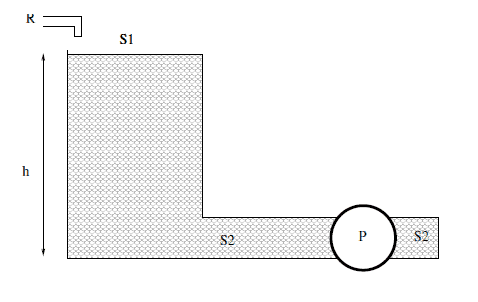# Pump used to halve the time taken by fluid to flow out

## Homework Statement

The tank in picture has section ##S_1## and it's linked with a tube of section ##S_2=\frac{1}{\sqrt{17}} S_1##. The tank is filled at an height ##h## and this level is kept constant by a sink ##R##, in such way that the exiting volumetric flow rate is ##Q=0.3 m^3/s## and constant. Determine the power ##P## of a pump located in the tube that can halve the time taken by the fluid to come out of the tank if the density of the fluid is ##\rho =1000kg/m^3##.## Homework Equations

Bernoulli equation

## The Attempt at a Solution

What is unclear to me is how to interpret the condition of halving the time taken by the fluid to come out. I interpeted it as equivalent to double the coming out speed of the fluid, that is to have a volumetric flow rate of ##2Q## instead of ##Q##. Is that possibly correct?

In that case I can write $$\rho g h (2Q) +P= \frac{1}{2} \rho (\frac{2Q}{S_2})^2 (2Q)$$ And find ##P## from here. Is the reasoning correct for this problem?

haruspex
Homework Helper
Gold Member

## Homework Statement

The tank in picture has section ##S_1## and it's linked with a tube of section ##S_2=\frac{1}{\sqrt{17}} S_1##. The tank is filled at an height ##h## and this level is kept constant by a sink ##R##, in such way that the exiting volumetric flow rate is ##Q=0.3 m^3/s## and constant. Determine the power ##P## of a pump located in the tube that can halve the time taken by the fluid to come out of the tank if the density of the fluid is ##\rho =1000kg/m^3##.
View attachment 102598

## Homework Equations

Bernoulli equation

## The Attempt at a Solution

What is unclear to me is how to interpret the condition of halving the time taken by the fluid to come out. I interpeted it as equivalent to double the coming out speed of the fluid, that is to have a volumetric flow rate of ##2Q## instead of ##Q##. Is that possibly correct?

In that case I can write $$\rho g h (2Q) +P= \frac{1}{2} \rho (\frac{2Q}{S_2})^2 (2Q)$$ And find ##P## from here. Is the reasoning correct for this problem?
I agree with your interpretation (of a poorly worded question). But note the information you have regarding S1. Think about how that might be relevant.

Last edited:
rude man
Homework Helper
Gold Member
What is unclear to me is how to interpret the condition of halving the time taken by the fluid to come out. I interpeted it as equivalent to double the coming out speed of the fluid, that is to have a volumetric flow rate of ##2Q## instead of ##Q##. Is that possibly correct?
I can think of no reasonable alternative interpretation. Sure!
In that case I can write $$\rho g h (2Q) +P= \frac{1}{2} \rho (\frac{2Q}{S_2})^2 (2Q)$$ And find ##P## from here. Is the reasoning correct for this problem?
You can write a second equation with P = 0 and eliminate h so that P = P(Q only).